Home > Misc

# Quantum dynamics of a classically chaotic system

$$\newcommand{\bm}{\mathbf} \newcommand{\bra}{\langle} \newcommand{\ket}{\rangle} \DeclareMathOperator{\sgn}{sgn}$$

The the first semester of 2003, I didn't know much quantum mechanics, and I knew even less about computational physics or numerically solving ordinary differential equations. In that semester I was enrolled in the fourth-year course "Advanced Hamiltonian Dynamics and Chaos", whose absurd course profile only listed second-year classical mechanics as a pre-requisite, but whose review of quantum mechanics included some fourth-year quantum optics.

While I eventually gained some understanding of the classical mechanics half of the course, I near-totally flopped on the quantum assignment – of the four parts to it, I did the first one adequately, grossly fudged the second, and made no attempt on parts 3 or 4. It was by far my worst large uni assignment. I thought it'd be interesting to revisit it, more than a decade on, and try to belatedly justify the (undeservedly high) passing grade I scraped for the course. While I'm vastly more competent at computational physics now than then, it had been over six years since I'd solved a physics problem of this size, so I had to go through a bit of re-learning. The end results are about as poor as they could be while still justifying the exercise – I have a much better idea of what was in that part of the course, and perhaps my results would be worth 15+ marks out of 20 if I wrote them up according to the marking criteria. An assignment earning 75% probably doesn't make for the greatest reading, but not many of you have read this far anyway.

The assignment studied a Hamiltonian that is an approximation to the one that was published in PRA last year (see Lenz et al., arXiv:1011.0242). Being a physics paper, it contains a lot of superfluous detail about experimental setups and SI units and so forth, but also a much more thorough analysis of the quantum tunnelling and its relation to the classical behaviour than what appears below. I just stick to what my assignment asked of me.

## Introduction, classical dynamics

The potential function used in Lenz et al. is $$V(q) = \lambda(1 + \varepsilon \cos(t))\sqrt{q^2 + 1}$$. We were told to analyse an approximation to this, the quantum Hamiltonian being

\begin{equation} \hat{H}(t) = \frac{\hat{p}^2}{2} + \lambda(1 + \varepsilon \cos(t))|\hat{q}|. \end{equation}

So, we have a V-shaped potential, the slope of which has magnitude $$\lambda$$ plus a periodic driving term. The classical version of this Hamiltonian results in the equations

\begin{align} \nonumber \dot{p} = -\frac{\partial H}{\partial q} &= -\lambda(1 + \varepsilon \cos(t))\sgn(q), \\ \dot{q} = \frac{\partial H}{\partial p} &= p. \end{align}

Fixing the parameters $$\lambda$$ and $$\varepsilon$$, we're left with three variables: $$p,\, q,\, t$$. Three-dimensional plots of the dynamics would be difficult to visualise, so we make a Poincaré section, leaving just $$q$$ and $$p$$ axes. We start at some location $$(q(0),\, p(0))$$, evolve the trajectory forward in time, but only plot points at integer multiples of the driving period. In regular motion, those points will (in the limit) form continuous contours; in chaotic motion, the points are scattered. By starting trajectories at a range of different places, we build up a picture of the possible dynamics in the system.

Here is the Poincaré section for $$\lambda = 1,\, \varepsilon = 0.3$$:(Despite the appearance caused by the axis ranges I plotted over, it's not the case that all the dynamics outside this window is chaotic – there are large regions at greater values of $$q$$ and $$p$$ showing regular behaviour.) I've deliberately drawn this asymmetrically, so that it's clear that there are two period-1 resonances visible as opposed to a single period-2 resonance; the small dot at around $$(0,\, 1.76)$$ is a fixed point.

There are two obvious candidates for fixed points in the Poincaré map. The first is the one I just pointed out: we can start at $$q=0,\, p=p_0 \neq 0$$, with $$p_0$$ chosen so that the particle returns to $$q=0$$ with $$p=p_0$$ after one driving period. Alternatively, we can start at $$q=q_0 \neq 0,\, p=0$$, with $$q_0$$ chosen so that the particle is at rest at $$q = q_0$$ after one driving period. In the Poincaré section above, I also started a trajectory near this latter fixed point, at around $$(1.04,\, 0)$$, but the fixed point is unstable, and the small early numerical errors meant that the trajectory was chaotically smeared out.

Perhaps there's a symmetry argument to explain the absence of further fixed points, but I haven't tried to formalise it, and I'm happy enough just to look at the Poincaré section.

The stable period-1 resonances of the classical system will be a focal point of the quantum dynamics, so it is worth deriving their positions. Let $$q(0) = 0$$ and $$\dot{q}(0_ = p_0 > 0$$ (the $$p_0 < 0$$ case is symmetrical). Until $$q(t)$$ passes zero again, it satisfies

\begin{equation} \ddot{q}(t) = -\lambda(1 + \varepsilon \cos(t)), \end{equation}

and hence

\begin{equation} \label{de_qdot} \dot{q}(t) = p_0 - \lambda(t + \varepsilon\sin(t)), \end{equation}

and hence

\begin{equation} \label{de_q} q(t) = p_0 t - \frac{1}{2}\lambda t^2 + \lambda\varepsilon\cos(t) - \lambda\varepsilon. \end{equation}

Let $$t'$$ be the first time greater than zero at which $$q(t) = 0$$. For $$t > t'$$ and until $$q(t)$$ reaches zero again, $$q(t)$$ satisfies

\begin{equation} \ddot{q}(t) = \lambda(1 + \varepsilon\cos(t)), \end{equation}

and hence

\begin{equation} \dot{q}(t) = \dot{q}(t') + \lambda(t + \varepsilon\sin(t) - t' - \varepsilon\sin(t')). \end{equation}

From (\ref{de_qdot}), we know that $$\dot{q}(t') = -\lambda(t' + \varepsilon\sin(t')) + p_0$$, so

\begin{equation} \dot{q}(t) = \lambda(t + \varepsilon\sin(t) - 2t' - 2\varepsilon\sin(t')) + p_0. \end{equation}

Since we're looking for a fixed point, we require that $$\dot{q}(\tau) = p_0$$. This requirement implies that

\begin{equation} 2t' + 2\varepsilon\sin(t') - \tau = 0, \end{equation}

which has the solution $$t' = \tau/2$$, which we could have arrived at by a symmetry argument. (The same doesn't hold true when finding a fixed point with $$q(0) \neq 0$$.) We can therefore substitute $$q(\tau/2) = 0$$ into (\ref{de_q}) and solve for the value of $$p_0$$ that gives a fixed point of the Poincaré map:

\begin{equation} \label{stable_fixedpt} p_0 = \lambda\left(\frac{4\varepsilon}{\tau} - \frac{\tau}{4}\right). \end{equation}

Here is some C code to output points in the Poincaré section; I have only compiled it under lcc-win32 but it looks pretty platform-independent to me.

/* Evolves (p(t), q(t)) according to a driven Hamiltonian;
outputs the Poincare section defined as the time being a
multiple of the driving period. */

#include <stdio.h>
#include <stdlib.h>
#include <math.h>

double tau = 6.28318530717959;

/* Parameters in the Hamiltonian */
double omega = 1.0;
double lambda = 1.0;
double epsilon = 0.3;

int sgn(double);
void calc_der(double[], double[], double);
double rrand(void);

int sgn(double x) {
int s;
s = (x > 0) - (x < 0);
return s;
}

void calc_der(double y[], double dydt[], double t) {
/* The DE system being solved */
dydt = -1.0*lambda*(1 + epsilon*cos(omega*t))*(double)sgn(y);
dydt = y;
}

double rrand() {
/* A rubbish random number generator on [0, 1] */
double x = (double)rand() / (double)RAND_MAX;
return x;
}

void main() {
long ct;

/* Array containing position and momentum: */
double pq;

/* Auxiliary arrays for the RK4: */
double k1;
double k2;
double k3;
double k4;
double vec_aux;

long max_index = 1;

srand(1234);

FILE *pq_out;
pq_out = fopen("poincare_pq.csv", "w");
fprintf(pq_out, "p,q\n");

long num_periods = 1000;
long steps_per_period = 1e5;
double h = (tau/omega) / (double)steps_per_period;
double t = 0.0;

double t_r;

long num_start_pts = 50;

for(long contour_ct=0; contour_ct < num_start_pts; contour_ct++) {
printf("Starting contour %d.\n", contour_ct);

/* Initial values p(0) and q(0): */
if (contour_ct == num_start_pts - 2) {
/* Try to hit the period-1 resonance with p=0 (Newton-Raphson) */
t_r = tau/4.0;
for(int j=0; j<5; j++) {
t_r = t_r - (0.5*tau - 2.0*(t_r + epsilon*sin(t_r))) / (-2.0*(1.0 + epsilon*cos(t_r)));
}

pq = 0.0;
pq = 0.5*lambda*t_r*t_r - lambda*epsilon*(cos(t_r) - 1.0);

printf("q_0 = %2.5e, for t' = %2.5e\n", pq, t_r);
} else if (contour_ct == num_start_pts - 1) {
/* Try to hit the period-1 resonance with q=0 */
pq = lambda*(4.0*epsilon/tau + tau/4.0);
pq = 0.0;

printf("p_0 = %2.5e\n", pq);
} else {
pq = rrand()*5.0 - 2.5;
pq = rrand()*5.0 - 2.5;
}

/* Print initial conditions: */
fprintf(pq_out, "%2.5f,%2.5f\n", pq, pq);

for(long period_ct=0; period_ct < num_periods; period_ct++) {
for (long step_ct=0; step_ct < steps_per_period; step_ct++) {
/* RK4: */
calc_der(pq, k1, t);

for(ct=0;ct<max_index+1;ct++) {
vec_aux[ct] = pq[ct] + 0.5*h*k1[ct];
}
calc_der(vec_aux, k2, t+0.5*h);

for(ct=0;ct<max_index+1;ct++) {
vec_aux[ct] = pq[ct] + 0.5*h*k2[ct];
}
calc_der(vec_aux, k3, t+0.5*h);

for(ct=0;ct<max_index+1;ct++) {
vec_aux[ct] = pq[ct] + h*k3[ct];
}
calc_der(vec_aux, k4, t+h);

for(ct=0;ct<max_index+1;ct++) {
pq[ct] = pq[ct] + h*(k1[ct] + 2.0*k2[ct] + 2.0*k3[ct] + k4[ct]) / 6.0;
}

t += h;
}

/* Output to file at the end of the driving period */
fprintf(pq_out, "%2.5f,%2.5f\n", pq, pq);
}
}
fclose(pq_out);
}

## Quantum dynamics

### Building the Hamiltonian matrix

The first assignment question asks for the first six (why six?) energy eigenstates and their eigenvalues of the undriven system $$\varepsilon = 0$$, helpfully suggesting that we use harmonic oscillator eigenstates as basis functions. Defining the Hermite polynomials by the physicists' convention,

\begin{equation} \label{hermite_defn} H_n(x) = (-1)^n \exp(x^2) \frac{d^n}{dx^n} \exp(-x^2), \end{equation}

the $$n$$th eigenfunction of the harmonic osillator is

\begin{equation} \label{sho_n} \hspace{-10pt} u_n(x) = \frac{1}{\sqrt{2^n n!}} \left(\frac{2m\omega}{\tau\hbar}\right)^{1/4} \exp\left(\frac{-m\omega x^2}{2\hbar}\right) H_n\left(\sqrt{\frac{m\omega}{\hbar}} x\right). \end{equation}

To make my life easier, I'll set $$m = \omega = \hbar = 1$$, leaving

\begin{equation} u_n(x) = \frac{1}{\sqrt{2^n n!}} \left(\frac{2}{\tau}\right)^{1/4} \exp(-x^2/2) H_n(x). \end{equation}

The matrix elements of the Hamiltonian are $$H_{nm} = \bra n|\hat{H}|m\ket$$. In the position representation, the potential term is just $$|x|$$ times the appropriate constant, and the kinetic term is $$-\frac{1}{2}\frac{d^2}{dx^2}$$. The matrix elements can then be evaluated numerically as

\begin{equation} \hspace{-10pt} H_{nm} = \int_{-\infty}^{\infty} u_n^*(x) \left(-\frac{1}{2}\frac{d^2}{dx^2} + \lambda(1 + \varepsilon\cos(t))|x|\right)u_m(x) dx. \end{equation}

But why make the computer spend a few minutes crunching the integrals when we can spend a few days solving them by hand? Firstly, we don't actually need integrals for the kinetic term – since we're working in a number-state basis, we can write $$\hat{p}^2$$ in terms of creation and annihilation operators and do things in a few lines. We recall that

\begin{align} \hat{a}|n\ket &= \sqrt{n}\,|n-1\ket, & \hat{a}^{\dagger}|n\ket &= \sqrt{n+1}\,|n+1\ket, \end{align}

and look up on Wikipedia that

\begin{equation} \hat{p} = \frac{-i}{\sqrt{2}} (\hat{a} - \hat{a}^{\dagger}), \end{equation}

so that

\begin{equation} \hat{p}^2 = -\frac{1}{2}(\hat{a}^2 + \hat{a}^{\dagger 2} - \hat{a}\hat{a}^{\dagger} - \hat{a}^{\dagger}\hat{a}). \end{equation}

After a couple of lines of algebra, we get

\begin{align} \nonumber \bra n|\frac{\hat{p}^2}{2}|m\ket &= -\frac{1}{4}(\sqrt{m(m-1)}\delta_{n,m-2} + \sqrt{n(n-1)}\delta_{n-2,m} \\ & \qquad \qquad -\, (2n+1)\delta_{nm}). \end{align}

The potential term is more difficult. Since the basis functions $$u_n(x)$$ are all real, we have integrals of the form

$\int_{-\infty}^{\infty} |x|u_n(x)u_m(x) dx.$

Now, $$u_n(x)$$ is an even function if $$n$$ is even and odd otherwise, and $$|x|$$ is even. So if $$n$$ and $$m$$ are of different parity, then the integrand is odd, and the integral will be zero. We therefore need only consider cases where $$n$$ and $$m$$ have the same parity, and in the rest of this section, I will leave this assumption unstated.

We can get rid of the pesky absolute value function by realising that the integral over the whole real axis is twice the integral over the positive real axis. Ignoring some constant terms that can be re-inserted at the end, the integral is

\begin{equation} \label{hermite_integral} I_{nm} = \int_0^{\infty} xH_n(x)H_m(x)\exp(-x^2) dx. \end{equation}

I couldn't find this integral in a couple of books of integral tables, so it's time for some exercises. To save a bit of space, I'll use $$D(f(x))$$ to denote $$df/dx$$. The first step is to substitute the definition (\ref{hermite_defn}) into one of the terms in (\ref{hermite_integral}). This gives

\begin{equation} I_{nm} = (-1)^m \int_0^{\infty} xH_n(x) D^m(\exp(-x^2)) dx. \end{equation}

The procedure now is to repeatedly integrate by parts, moving the derivatives from $$\exp(-x^2$$ onto $$xH_n(x)$$. Without loss of generality, we can assume that $$n \le m$$. If $$n = m$$, then we'll be left with an integrand of $$x\exp(-x^2)$$ along with a collection of boundary terms; if $$n \le m-2$$, then we'll just have the collection of boundary terms, since eventually we'll be taking the derivative of a constant.

I'll only sketch the details, because life's too short to type up the full thing. We'll start with the Hermite polynomial identity

\begin{equation} D(H_n(x)) = 2nH_{n-1}(x). \end{equation}

With this result and a bit of induction, we can show that

\begin{align} \nonumber D^k(xH_n(x)) &= k \cdot 2^{k-1} \frac{n!}{(n-k+1)!} H_{n-k+1}(x) \\ \label{xhermite_derivs} & \qquad +\, 2^k \frac{n!}{(n-k)!} xH_{n-k}(x). \end{align}

These derivatives will mostly show up as boundary terms, evaluated at $$x=0$$ (the exponential ensures no boundary terms at infinity). The $$xH_{n-k}(x)$$ term will always vanish at $$x=0$$, but $$H_{n-k+1}(0)$$ is non-zero for $$n-k+1$$ even. From

\begin{equation} H_{2n}(0) = (-1)^n \frac{(2n)!}{n!}, \end{equation}

we get

\begin{equation} \label{boundary_term_1}\hspace{-10pt} D^k(xH_n(x))\vert_{x=0} = \begin{cases} \frac{(-1)^{(n-k+1)/2} k\cdot 2^{k-1} n!}{[\frac{1}{2}(n-k+1)]!} & \text{if } n-k \text{ odd} \\ 0 & \text{otherwise.} \end{cases} \end{equation}

Even derivatives of the exponential also contribute to the boundary terms:

\begin{equation} \label{boundary_term_2} D^{2n}(\exp(-x^2)) = (-1)^n \frac{(2n)!}{n!}. \end{equation}

The boundary terms form a sum

\begin{equation} \label{boundary_term_sum} S_l = (-1)^m \sum_{k=0}^l \left. (-1)^k D^k\left[xH_n(x)\right] D^{m-k-1}[\exp(-x^2)]\right|_{x=0}. \end{equation}

For $$n=m$$, one non-boundary-term contributes to the integral, the overall result being

\begin{equation} I_{nm} = S_{n-1} + 2^{n-1}(n+1)!. \end{equation}

For $$n \le m-2$$, we only have boundary terms, and

\begin{equation} I_{nm} = S_{n+1}. \end{equation}

Substituting (\ref{boundary_term_1}) and (\ref{boundary_term_2}) into (\ref{boundary_term_sum}) gives terms that look like

\begin{equation} s_{nmk} = \frac{(-1)^{(n+m)/2}\, k\cdot 2^{k-1} n! (m-k-1)!}{[\frac{1}{2}(n-k+1)]![\frac{1}{2}(m-k-1)]!}. \end{equation}

And with this space-saving notation, we have our integral (\ref{hermite_integral}):

\begin{equation} I_{nm} = \begin{cases} \phantom{-}\sum_{k=0,\text{even}}^{n-1} s_{nmk} + 2^{n-1}(n+1)! & \text{if } n=m \text{ odd} \\ -\sum_{k=1,\text{odd}}^{n-1} s_{nmk} + 2^{n-1}(n+1)! & \text{if } n=m \text{ even} \\ \phantom{-}\sum_{k=0,\text{even}}^{n+1} s_{nmk} & \text{if } n<m \text{ both odd} \\ -\sum_{k=1,\text{odd}}^{n+1} s_{nmk} & \text{if } n<m \text{ both even} \\ \phantom{-}0 & \text{otherwise.} \end{cases} \end{equation}

Whew! These values need to be multiplied by both 2 and the various normalisation factors in (\ref{sho_n}) before becoming part of the Hamiltonian matrix $$H_{nm}$$, but I'll leave those minor details to my Octave code below. I started writing most of what follows in C, and didn't bother vectorising some of the for loops. I've also been lazy and left some augmented assignments in place; the probability of a MATLAB user wanting to run my code is even lower than the probability of someone having read this far, but for that empty set of people, you'll have to either switch to Octave or fix the "-=" lines.

% quantum_setup.m
%
% Code to prepare matrices in the Hamiltonian, grids
% in position- and momentum-space, harmonic oscillator
% basis wavefunctions, a function to calculate <x>,
% <x^2>, etc.
%
% Some of the calculations are done in log terms to avoid
% overflows with the factorials; this isn't necessary for
% small dimensions of the (truncated) Hilbert space.

clear

global tau = 6.28318530717959;

% Parameters in the Hamiltonian:
omega = 1.0;

steps_per_period = 1e4;
dt = (tau/omega) / steps_per_period;

steps_per_sample = 1e2;
steps_per_xp_sample = 1e2;

% Number of harmonic oscillator number states to
% use as the (truncated) basis of the Hilbert space:
global N_basis = 20;

% Odds and evens refer to odd and even basis functions,
% which are indexed from 0.  Octave vectors are indexed
% from 1, hence the backwards-looking definitions.
odds = 2:2:N_basis;
evens = 1:2:N_basis;

% Main grid is in position-space:
x_min = -10;
x_max = 10;
nx_grid = 128;

dx = (x_max - x_min)/(nx_grid - 1);
% The offset makes x "FFT-even", which was handy while
% I was re-working-out how FFT's work....
x = (x_min-dx/2):dx:(x_max-dx/2);

% Momentum grid is Fourier space:
dp = tau/(nx_grid*dx);
p1 = 0:dp:((ceil(nx_grid/2)-1)*dp);
np_grid_leftover = nx_grid - length(p1);
p2 = (-(np_grid_leftover)*dp):dp:-dp;
p = [p1, p2];
p_shift = fftshift(p);

function log_fact = log_factorial(n)
log_fact = 0.0;
if (n > 1)
j = 2:n;
log_fact = sum(log(j));
end
endfunction

function h = dim_hermite(n, x)
% Calculates exp(-x^2/2) * H_n(x) (a "diminished Hermite polynomial").

switch(n)
case (0)
h = exp(-0.5*x.*x);
case (1)
h = 2.0*x.*exp(-0.5*x.*x);
otherwise
h = 2.0*x.*dim_hermite(n-1, x) - 2.0*(n - 1.0)*dim_hermite(n-2, x);
endswitch
endfunction

function psi = calc_sho_n(n, x)
% Simple harmonic oscillator basis wavefunction.

global tau;

sign = 1.0;
herm = dim_hermite(n, x);
if (herm < 0)
herm = -1.0*herm;
sign = -1.0;
end

log_psi = -n/2.0*log(2.0) - 0.5*log_factorial(n) - 0.25*log(tau) + 0.25*log(2.0) + log(herm);
psi = sign*exp(log_psi);
endfunction

% Calculate basis wavefunctions in position-
% and momentum-space:

psi_basis_x = zeros(N_basis, nx_grid);
psi_basis_p = zeros(N_basis, nx_grid);

for n = 0:(N_basis - 1)
fprintf("Starting basis function n = %d\n", n);

psi_n_x = calc_sho_n(n, x);
psi_n_p = fftshift(fft(psi_n_x));

psi_basis_x(n+1, :) = psi_n_x;
psi_basis_p(n+1, :) = psi_n_p;
end

% Matrix elements of p^2:
p_sq = zeros(N_basis);

for j = 0:(N_basis - 1)
for k = 0:(N_basis - 1)
if (k == j-2)
p_sq(j+1, k+1) = -0.5 * sqrt(j*(j-1));
p_sq(k+1, j+1) = -0.5 * sqrt(j*(j-1));
end

if (k == j)
p_sq(j+1, k+1) = 0.5 * (2*j + 1);
end
end
end

% Matrix elements of |q|; uses a couple of auxiliary functions.
V = zeros(N_basis);

function p = parity(n)
p = mod(n, 2);
endfunction

function log_fact = log_factorial(n)
log_fact = 0.0;
if (n > 1)
j = 2:n;
log_fact = sum(log(j));
end
endfunction

function s = sum_terms(k, n, m)
global tau
s = log(k) + (k-1.0)*log(2.0) + log_factorial(n) + log_factorial(m-k-1);
s -= log_factorial((n-k+1)/2) + log_factorial((m-k-1)/2);

% Normalisation terms from the full basis wavefunctions:
s -= 0.5*n*log(2.0) + 0.5*log_factorial(n) + 0.25*log(tau) - 0.25*log(2.0);
s -= 0.5*m*log(2.0) + 0.5*log_factorial(m) + 0.25*log(tau) - 0.25*log(2.0);

s = exp(s);
endfunction

for m = 0:(N_basis - 1)
for n = 0:m
sum_s = 0;

if (parity(n) == parity(m))
k_max = n + 1;

if (n == m)
k_max = n - 1;
log_extra_term = (n - 1.0)*log(2.0) + log_factorial(n+1);
% Normalisation terms from the full basis wavefunctions:
log_extra_term -= n*log(2.0) + log_factorial(n) + 0.5*log(tau) - 0.5*log(2.0);
sum_s = exp(log_extra_term);
end

sum_sign = -1;
if (parity((n+m)/2) == 0)
sum_sign = 1;
end

k_min = 0;
if (parity(n) == 0)
k_min = 1;
sum_sign = -1*sum_sign;
end

for k = k_min:2:k_max
sum_s = sum_s + sum_sign * sum_terms(k, n, m);
end

sum_s = 2.0*sum_s;
end
V(n+1,m+1) = sum_s;
V(m+1,n+1) = sum_s;
end
end

function expec_vals = avg_xp(psi)
% Input is a vector in the number-state basis.
%
% Returns a vector:
% avg_xp(1) = <x>
% avg_xp(2) = <x^2> - <x>^2
% avg_xp(3) = <p>
% avg_xp(4) = <p^2> - <p>^2
%
% Opaque-looking formulas come from writing, e.g.,
%    x = (a + a-dagger)/sqrt(2)
% and simplifying the double-sum in
%    <psi|x|psi>
% when you write |psi> in the number-state basis.

expec_vals = zeros(4, 1);

N = length(psi);
n = (0:(N-1))';

expec_vals(1) = sqrt(2) * sum(sqrt(n(2:N)) .* real(psi(2:N) .* conj(psi(1:(N-1)))));

expec_x2 = sum((n+0.5).*abs(psi).^2) + sum(sqrt((n(1:(N-2)) + 1) .* (n(1:(N-2)) + 2)) .* real(conj(psi(1:(N-2))) .* psi(3:N)));
expec_vals(2) = expec_x2 - expec_vals(1)^2;

expec_vals(3) = sqrt(2) * sum(sqrt(n(2:N)) .* imag(psi(2:N) .* conj(psi(1:(N-1)))));

expec_p2 = sum((n+0.5).*abs(psi).^2) - sum(sqrt((n(1:(N-2)) + 1) .* (n(1:(N-2)) + 2)) .* real(conj(psi(1:(N-2))) .* psi(3:N)));
expec_vals(4) = expec_p2 - expec_vals(3)^2;
endfunction

### Some dynamics with $$\varepsilon = 0$$

With the matrices ready, we can start calculating things. Here are the first six energy eigenvalues and eigenstates: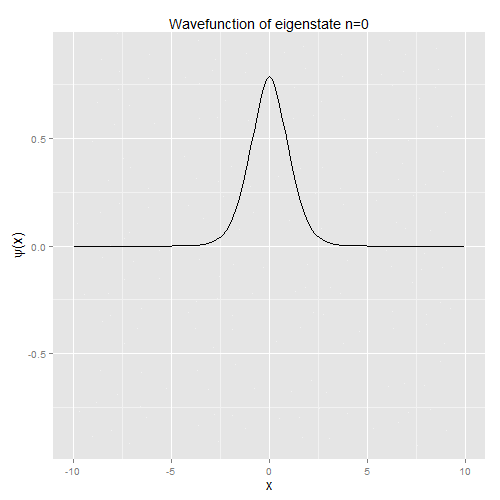The first few eigenstates have a large overlap with the corresponding harmonic oscillator number state. Letting $$|E_n\ket$$ be the $$n$$th energy eigenstate, the overlaps $$|\bra n|E_n\ket|^2$$ decrease: 0.998, 0.985, 0.94, 0.82, 0.64, 0.40, and soon the overlaps are very small. Which makes sense – the potential rises slower than a quadratic, and the gap between consecutive energy eigenvalues falls.

Octave code:

% Computes eigenvalues and eigenstates of the Hamiltonian
% H = (1/2)p^2 + V
% with V calculated in quantum_setup.m.

quantum_setup

H = 0.5*p_sq + V;

[evecs, evals] = eig(H);

evals = diag(evals);
[evals, idx] = sort(evals);

out_file = fopen("eigenstates/evals.csv", "w");
fprintf(out_file, "Energy_level,energy_eigenvalue\n");
for ct = 1:6
fprintf(out_file, "%d,%2.5e\n", ct-1, evals(idx(ct)));
end
fclose(out_file);

for ct = 1:6
out_file_str = sprintf("eigenstates/%d.csv", ct-1);
out_file = fopen(out_file_str, "w");
fprintf(out_file, "x,psi(x)\n");
psi = evecs(:, idx(ct));
psi_x = transpose(psi) * psi_basis_x;

for j = 1:nx_grid
fprintf(out_file, "%2.5e,%2.5e\n", x(j), psi_x(j));
end

fclose(out_file);
end

An initial harmonic oscillator coherent state doesn't remain as a Gaussian-ish wavepacket for long:The expectation value of position and momentum over time (dashed lines are at $$\bra \hat{x}\ket \pm \sqrt{\bra \hat{x}^2\ket - \bra \hat{x}\ket^2}$$ and similarly for $$\hat{p}$$):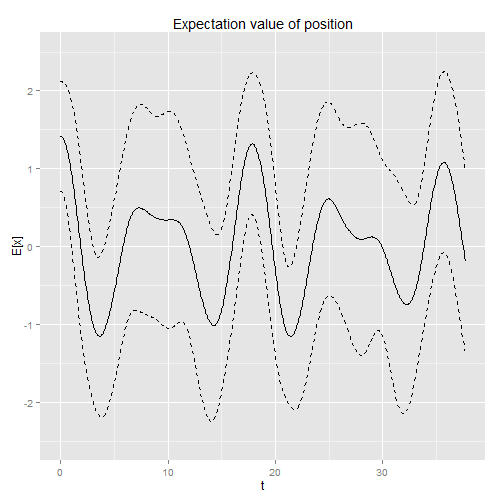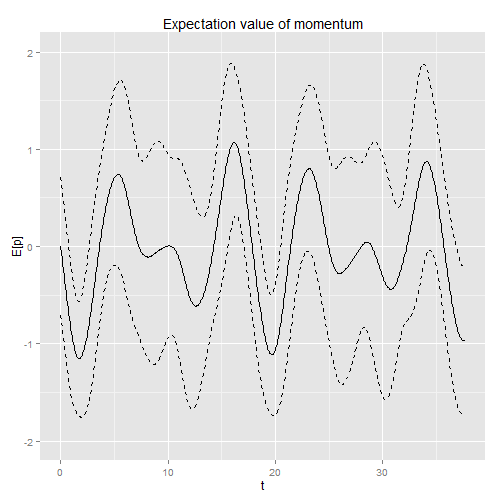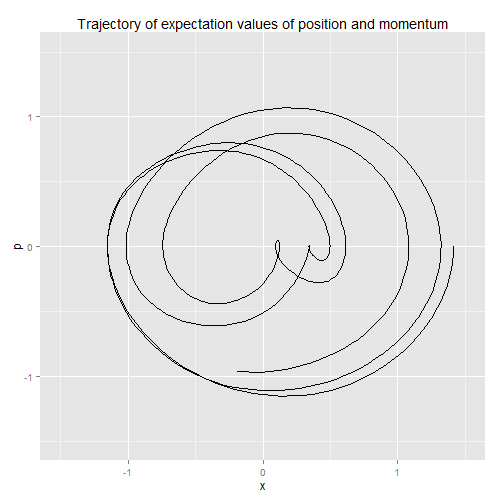Octave code:

% quantum_rk4.m
% RK4 solver for Schroedinger's equation;
% H = 0.5*p_sq + [parameters]*V, with p_sq and V calculated in
% quantum_setup.m

quantum_setup

num_periods = 6;

lambda = 1;
epsilon = 0.0;

% Coherent state:
% <q> = 0, <p> on period-1 resonance:
% alpha = i*lambda*(4.0*epsilon/tau + tau/4.0)/sqrt(2.0);
alpha = 1;

% Set up coherent states:
r = abs(alpha);
phase = arg(alpha);
psi = zeros(N_basis, 1);

% Following will fail if r = 0.
for j = 0:(N_basis - 1)
log_mag = -1.0*r*r/2.0 + j*log(r) - 0.5*log_factorial(j);
new_phase = j*phase;
psi(j+1) = exp(log_mag + i*new_phase);
end

t = 0;
output_ct = 0;

avg_xp_out = fopen("rk4_outputs/avg_xp.csv", "w");
fprintf(avg_xp_out, "t,E_x,Var_x,E_p,Var_p\n");

for ct_period = 1:num_periods
for ct_step = 1:steps_per_period
if (mod(ct_step-1, steps_per_sample) == 0)
% x-space:
outfile_name = sprintf("rk4_outputs/%d.csv", output_ct);
file_out = fopen(outfile_name, "w");
fprintf(file_out, "x,mod_psi_sq,t %f\n", t);

psi_x = transpose(psi) * psi_basis_x;

for ct = 1:nx_grid
fprintf(file_out, "%2.5e,%2.5e\n", x(ct), abs(psi_x(ct)).^2);
end
fclose(file_out);

%p-space:
outfile_name = sprintf("rk4_outputs/p_space_%d.csv", output_ct);
file_out = fopen(outfile_name, "w");
fprintf(file_out, "p,mod_psi_sq,t %f\n", t);

psi_p = transpose(psi) * psi_basis_p;

mod2_psi_p = abs(psi_p).^2;
normalisation = sum(mod2_psi_p);

for ct = 1:nx_grid
fprintf(file_out, "%2.5e,%2.5e\n", p_shift(ct), abs(psi_p(ct)).^2/normalisation);
end
fclose(file_out);

output_ct = output_ct + 1;
end

if (mod(ct_step-1, steps_per_xp_sample) == 0)
moments = avg_xp(psi);
fprintf(avg_xp_out, "%2.5e,%2.5e,%2.5e,%2.5e,%2.5e\n", t, moments(1), moments(2), moments(3), moments(4));
end

% RK4:
k1 = -i*(0.5*p_sq + lambda*(1 + epsilon*cos(t))*V)*psi;

psi_aux = psi + 0.5*dt*k1;
k2 = -i*(0.5*p_sq + lambda*(1 + epsilon*cos(t+0.5*dt))*V)*psi_aux;

psi_aux = psi + 0.5*dt*k2;
k3 = -i*(0.5*p_sq + lambda*(1 + epsilon*cos(t+0.5*dt))*V)*psi_aux;

psi_aux = psi + dt*k3;
k4 = -i*(0.5*p_sq + lambda*(1 + epsilon*cos(t+dt))*V)*psi_aux;

psi = psi + dt*(k1 + 2*k2 + 2*k3 + k4)/6;

t = t + dt;
end
end

% Final outputs:
% x-space:
outfile_name = sprintf("rk4_outputs/%d.csv", output_ct);
file_out = fopen(outfile_name, "w");
fprintf(file_out, "x,mod_psi_sq,t %f\n", t);

psi_x = transpose(psi) * psi_basis_x;

for ct = 1:nx_grid
fprintf(file_out, "%2.5e,%2.5e\n", x(ct), abs(psi_x(ct)).^2);
end
fclose(file_out);

%p-space:
outfile_name = sprintf("rk4_outputs/p_space_%d.csv", output_ct);
file_out = fopen(outfile_name, "w");
fprintf(file_out, "p,mod_psi_sq,t %f\n", t);

psi_p = transpose(psi) * psi_basis_p;

% Some Fourier-transform normalisation I haven't
% worked out:
mod2_psi_p = abs(psi_p).^2;
normalisation = sum(mod2_psi_p);

for ct = 1:nx_grid
fprintf(file_out, "%2.5e,%2.5e\n", p_shift(ct), abs(psi_p(ct)).^2/normalisation);
end
fclose(file_out);

moments = avg_xp(psi);
fprintf(avg_xp_out, "%2.5e,%2.5e,%2.5e,%2.5e,%2.5e\n", t, moments(1), moments(2), moments(3), moments(4));
fclose(avg_xp_out);

### Some dynamics with $$\varepsilon \neq 0$$

And now we reach the main goal of the assignment, and amusingly enough, I start to lose the thread a little. I'll start with the bits that I have figured out, which at least represents an advance on where I was 11 years ago.

With a time-independent Hamiltonian, we can represent the Hamiltonian as a constant matrix, and find its eigenvalues and eigenvectors, and so forth, as was done above. When the Hamiltonian is time-dependent, that's no longer true. In the case of a preiodically-driven Hamiltonian, as we have with $$V(\hat{q}) = \lambda(1 + \varepsilon\cos(t))|\hat{q}|$$, we can get around this problem by calculating the operator that evolves an arbitrary state $$|\psi(t)\ket$$ to $$|\psi(t+T)\ket$$, where $$T$$ is the driving period, which with a $$\cos(t)$$ driving term is just $$\tau$$. This operator is called the Floquet operator, and is denoted $$\hat{F}$$:

\begin{equation} \hat{F}|\psi(t)\ket = |\psi(t+\tau)\ket. \end{equation}

It is essentially the quantum analogue to the Poincaré map, but as we'll see a little way below, its eigenvalues act in a way analogous to the eigenvalues of a time-independent Hamiltonian.

How do we calculate the Floquet operator? A better question might be, how do I calculate the Floquet operator? (The method I used might actually be the most common one, but it is somewhat displeasing.) For a time-independent Hamiltonian, we have a time-evolution operator

\begin{align} \nonumber \hat{U}(t')|\psi(t)\ket &= \exp(-i\hat{H}t'/\hbar)|\psi(t)\ket \\ &= |\psi(t+t')\ket. \end{align}

In our time-dependent system, we'll expand the exponential to first-order and hope that it's all valid to first order. That is (disregarding $$\hbar$$),

\begin{equation} |\psi(t + \Delta t)\ket \approx (1 - i\hat{H}(t)\Delta t)|\psi(t)\ket. \end{equation}

I'll use the notation

\begin{equation} \hat{U}_t(\Delta t) = 1 - i\hat{H}(t)\Delta t. \end{equation}

We can then chain these operators together:

\begin{align} \nonumber |\psi(t + 2\Delta t)\ket &\approx \hat{U}_{t + \Delta t}(\Delta t)|\psi(t + \Delta t)\ket \\ &\approx \hat{U}_{t + \Delta t}(\Delta t)\hat{U}_t(\Delta t)|\psi(t)\ket. \end{align}

So, multiply enough of those $$\hat{U}_{t + n\Delta t}(\Delta t)$$ matrices together, and we'll eventually get an approximation to the Floquet operator, evolving a state forward one driving period in time. It's distasteful to use an explicit numerical method, and the calculated matrix is "larger" than unitary (i.e., the diagonal elements of $$\hat{F}^{\dagger}\hat{F}$$ are greater than 1), but with sufficiently small values of $$\Delta t$$, things work OK.

The two tasks for this half of the assignment are 1) to find some eigenvectors and eigenvalues of the Floquet operator corresponding to regular and chaotic motion, and 2) to search for a case where an initial harmonic oscillator coherent state tunnels between the period-1 resonances of the classical system. I don't know what to make of the first part: for various eigenstates $$|\phi_n\ket$$, do we just find $$\bra\phi_n|\hat{x}|\phi_n\ket$$ and $$\bra\phi_n|\hat{p}|\phi_n\ket$$ and see whether the corresponding point $$x,\, p$$ in the classical Poincaré is in a regular or chaotic region?

The tunnelling part I'm more comfortable with. First, some background on tunnelling in a double-well potential. Let $$V(\hat{x}) = \hat{x}^4 - 4\hat{x}^2$$. The Hamiltonian has a near-degenerate pair of eigenvalues forming the ground state and first excited state: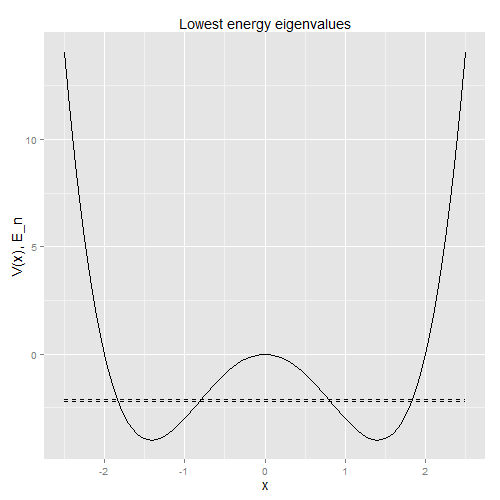Both the ground state and excited state wavefunctions are evenly spread in modulus-squared over each of the two wells, but the (not-modulus-squared) wavefunction of the ground state is even, and the wavefunction for the excited state is odd. So, a superposition of the two can be localised over one well or the other, depending on whether the states are added together, or one is subtracted from the other: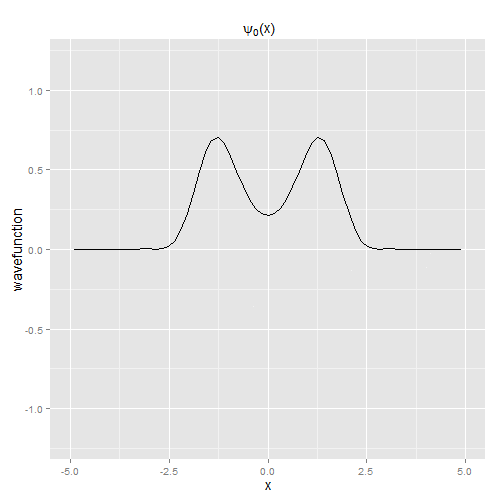Let $$|E_0\ket$$ and $$|E_1\ket$$ be the ground and excited states respectively, and let $$|\psi(0)\ket = \frac{1}{\sqrt{2}}(|E_0\ket + |E_1\ket)$$. This state evolves as

\begin{align} \nonumber |\psi(t)\ket &= \frac{1}{\sqrt{2}}(\exp(-iE_0t)|E_0\ket + \exp(-iE_1t)|E_1\ket) \\ &= \frac{1}{\sqrt{2}}\exp(-iE_0t)(|E_0\ket + \exp(-i(E_1 - E_0)t)|E_1\ket. \end{align}

When $$(E_1 - E_0)t = \tau/2$$, the state has evolved into the opposite superposition from which it started. That is, it has tunnelled from one well to the other: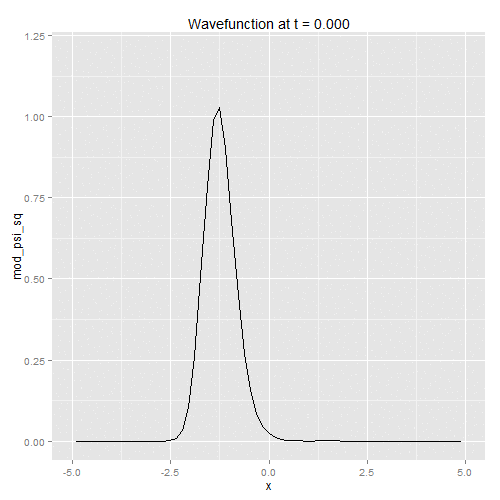Tunnelling between period-1 resonances of the driven Hamiltonian proceeds along similar lines. Eigenstates of the Floquet operator are either even or odd (waffle waffle, Hamiltonian has $$x \rightarrow -x$$ and $$p \rightarrow -p$$ symmetry, so commutes with the parity operator, which has eigenvalues of +1 and -1, so all energy eigenstates fall into either the +1 parity class or the -1 parity class, but I haven't got the details set into my head). We're told to start with a harmonic oscillator coherent state localised on a classical period-1 resonance; so call this state $$|\alpha\ket$$. We want to find even and odd eigenvectors $$|\phi_e\ket$$ and $$\phi_o\ket$$ of the Floquet operator such that, up to an overall phase and for some $$\theta$$,

\begin{equation} |\alpha\ket \approx \frac{1}{\sqrt{2}}(|\phi_e\ket + \exp(i\theta)|\phi_o\ket). \end{equation}

Now, since the Floquet operator is unitary, its eigenvalues lie on the unit circle and hence are of the form $$\exp(i\phi_n)$$. Applying $$\hat{F}$$ to the initial state gives

\begin{equation} \hat{F}|\alpha\ket \approx \frac{1}{\sqrt{2}}(\exp(i\phi_e)|\phi_e\ket + \exp(i\theta + i\phi_o)|\phi_o\ket), \end{equation}

and more generally,

\begin{align} \nonumber \hat{F}^k|\alpha\ket &\approx \frac{1}{\sqrt{2}}(\exp(ik\phi_e)|\phi_e\ket + \exp(i\theta + ik\phi_o)|\phi_o\ket) \\ &\approx \frac{1}{\sqrt{2}}\exp(ik\phi_e)(|\phi_e\ket + \exp(i[\theta + k(\phi_o - \phi_e)])|\phi_o\ket). \end{align}

If $$k(\phi_o - \phi_e) = \tau/2$$, then the superposition is (approximately) the opposite of the one we started with. The procedure is therefore:

• Create state $$|\alpha\ket$$ so that $$\bra\alpha|\hat{p}|\alpha\ket$$ is the $$p_0$$ of (\ref{stable_fixedpt}), and $$\bra\alpha|\hat{x}|\alpha\ket = 0$$.
• Find the even and odd eigenvectors of $$\hat{F}$$ which have the largest overlap with $$|\alpha\ket$$, and find $$\phi_e - \phi_o$$.
• Repeat for various choices of the parameters $$\lambda$$ and $$\varepsilon$$ in the Hamiltonian, until we find a $$(\phi_e - \phi_o)$$ which satisfies $$k(\phi_e - \phi_o) \approx \tau/2$$.

More generally you could loop over a fine grid of the parameter space and create a raster plot or something, but I don't want to work my poor laptop too hard.

The parameter values $$\lambda = 1.2,\, \varepsilon = 0.31$$ are the best I found with a not-too-thorough search of the parameter space. The overlap of the even eigenvector with $$|\alpha\ket$$ is around 0.57 (a little less than $$1/\sqrt{3}$$, hopefully close enough to $$1/\sqrt{2}$$, the overlap with the odd eigenvector is also around 0.57, and $$3(\phi_e - \phi_o) \approx 1.001\tau/2$$, so we should see tunnelling from one period-1 resonance to the other in three driving periods.

To check that these results aren't caused by a bug in the Floquet code, I ran the quantum_rk4 code with those values of $$\lambda$$ and $$\varepsilon$$ and the appropriate initial coherent state. The following gif shows the (mod-square) wavefunction in momentum-space at $$t=0,\, 3\tau,\, 6\tau$$.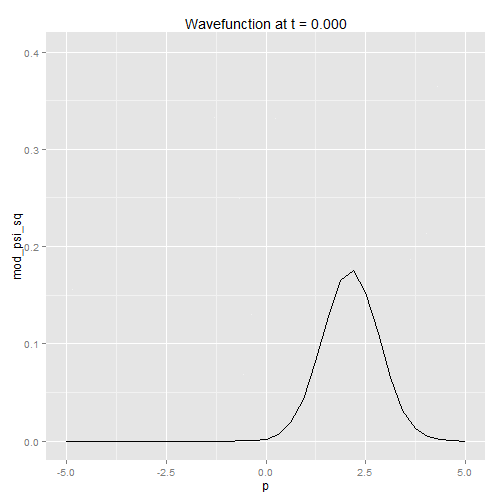It's a bit rough, it doesn't really tunnel to a state symmetrical to the initial state, so perhaps I've missed some key concept somewhere, but it's close enough for me. Whereas earlier we saw quantum behaviour that was clearly not classical, now we see quantum behaviour that is "very non-classical".

Octave code to calculate the Floquet operator and its eigenvalues etc. (a value of steps_per_period of 1e6, set in the quantum_setup.m file, results in a Floquet matrix that is unitary to within 1%):

% Calculates the Floquet operator for a Hamiltonian.
% Explicit method, just does U_t(dt) = I - iH*dt
% and multiplies all the U_t's together.

quantum_setup

file_out_str = sprintf("floq_outputs/evals_etc.csv");
file_out = fopen(file_out_str, "w");
fprintf(file_out, "lambda,epsilon,even_phase,odd_phase,2*delta_phase/tau,mod_overlap_even,mod_overlap_odd\n");

for lambda = 1.2
fprintf("Starting lambda = %2.3f\n", lambda);
for epsilon = 0.3:0.01:0.33
fprintf("Starting epsilon = %2.3f\n", epsilon);

t = 0;
% F will contain the Floquet matrix.
F = eye(N_basis);
Id = eye(N_basis);

tic

for ct_step = 1:steps_per_period
H = 0.5*p_sq + lambda*(1 + epsilon*cos(t))*V;
U = Id - i*dt*H;
F = U*F;
t = t + dt;
end

toc;

unitary_check = diag(F'*F);

% Coherent state |alpha>.
% <q> = 0, <p> on period-1 resonance:
alpha = i*lambda*(4.0*epsilon/tau + tau/4.0)/sqrt(2.0);

% Set up coherent state:
r = abs(alpha);
phase = arg(alpha);
psi = zeros(N_basis, 1);

% Following will fail if r = 0.
for j = 0:(N_basis - 1)
log_mag = -1.0*r*r/2.0 + j*log(r) - 0.5*log_factorial(j);
new_phase = j*phase;
psi(j+1) = exp(log_mag + i*new_phase);
end

[evecs, evals] = eig(F);
evals = diag(evals);
evals_phase = arg(evals);

% Want to separate even and odd Floquet eigenvectors.  Even
% eigenvectors will have large values in the "even" indices,
% similarly for the odd eigenvectors.

even_sums = sum(abs(evecs(evens, :)));
even_indices = find(even_sums > 0.5);

odd_sums = sum(abs(evecs(odds, :)));
odd_indices = find(odd_sums > 0.5);

even_evecs = evecs(:, even_indices);
odd_evecs = evecs(:, odd_indices);

% Find the overlaps of the eigenvectors with the initial
% coherent state; extract the largest overlap of the even
% eigenvectors and the largest overlap of the odd eigenvectors.
overlaps = (psi'*evecs)';
mod_overlaps = abs(overlaps);

mod_overlaps_even = mod_overlaps;
mod_overlaps_even(odd_indices) = 0;
max_even_overlap_index = find(mod_overlaps_even == max(mod_overlaps_even));

mod_overlaps_odd = mod_overlaps;
mod_overlaps_odd(even_indices) = 0;
max_odd_overlap_index = find(mod_overlaps_odd == max(mod_overlaps_odd));

eval_even = evals(max_even_overlap_index);
eval_odd = evals(max_odd_overlap_index);

% Find the difference in phase between the two chosen eigenvalues.
% Calculated by a dot product of vectors to avoid issues with angles
% going past tau/2 or whatever.
dot_evals = real(eval_even)*real(eval_odd) + imag(eval_even)*imag(eval_odd);
delta_phase = acos(dot_evals / (abs(eval_even) * abs(eval_odd)));

fprintf(file_out, "%2.5e,%2.5e,%2.5e,%2.5e,%2.5e,%2.5e,%2.5e\n",
lambda,
epsilon,
arg(evals(max_even_overlap_index)),
arg(evals(max_odd_overlap_index)),
2*delta_phase/tau,
abs(overlaps(max_even_overlap_index)),
abs(overlaps(max_odd_overlap_index)));
end
end

fclose(file_out);

## Conclusions

This was an interesting exercise. Generating the classical Poincaré section took a couple of hours, a substantial improvement on the couple of months that it took me back in 2003. An improvement shouldn't be too surprising, since I spent much of 2005 tracking fixed points of Poincaré maps, but I was surprised at just how much better I am at it now. The quantum stuff, on the other hand.... I ran into difficulties at a lot of steps, either trying to understand the lecture notes (for the first time!) or ironing out bugs in my code. The weird thing is that, now that at least some of the concepts are in my head, it doesn't seem particularly difficult. And indeed, the Floquet operator only took up a week of the lectures.

I certainly wouldn't say that I want to go back to physics research – occasionally the coding frustrations brought back the feelings of why I quit physics in the first place – but it was nice to be back in undergrad-physics-assignment land.

Posted 2014-02-09.

Home > Misc